Question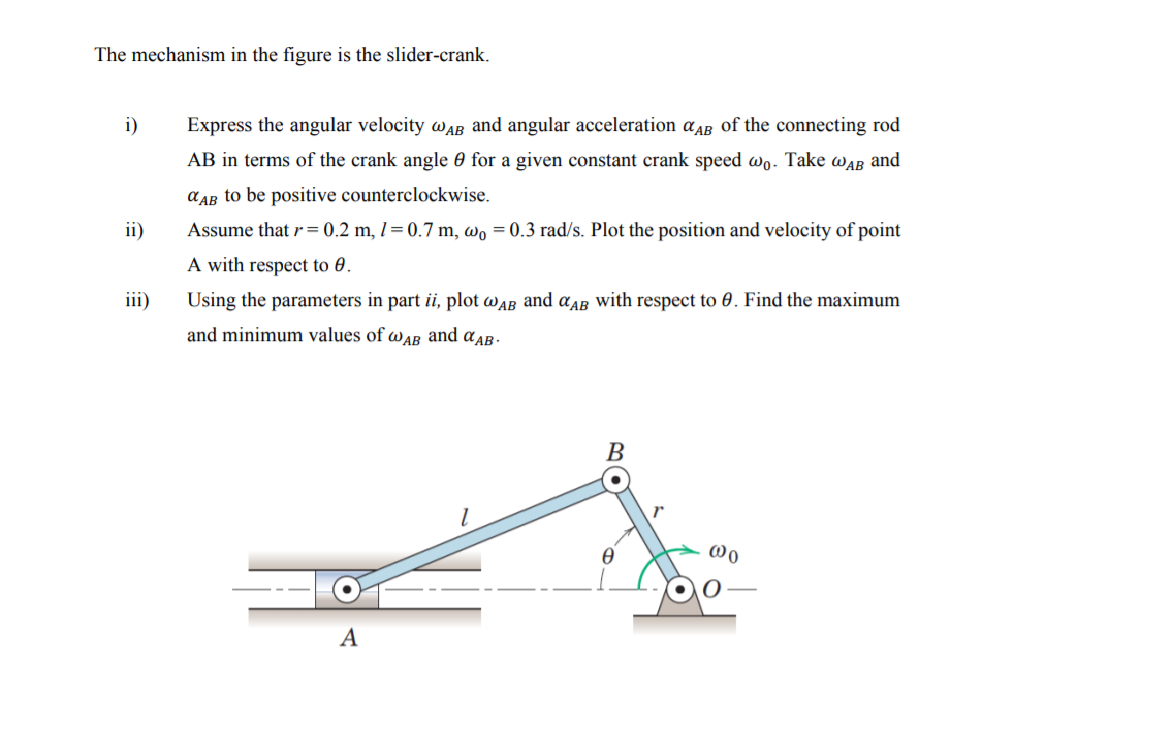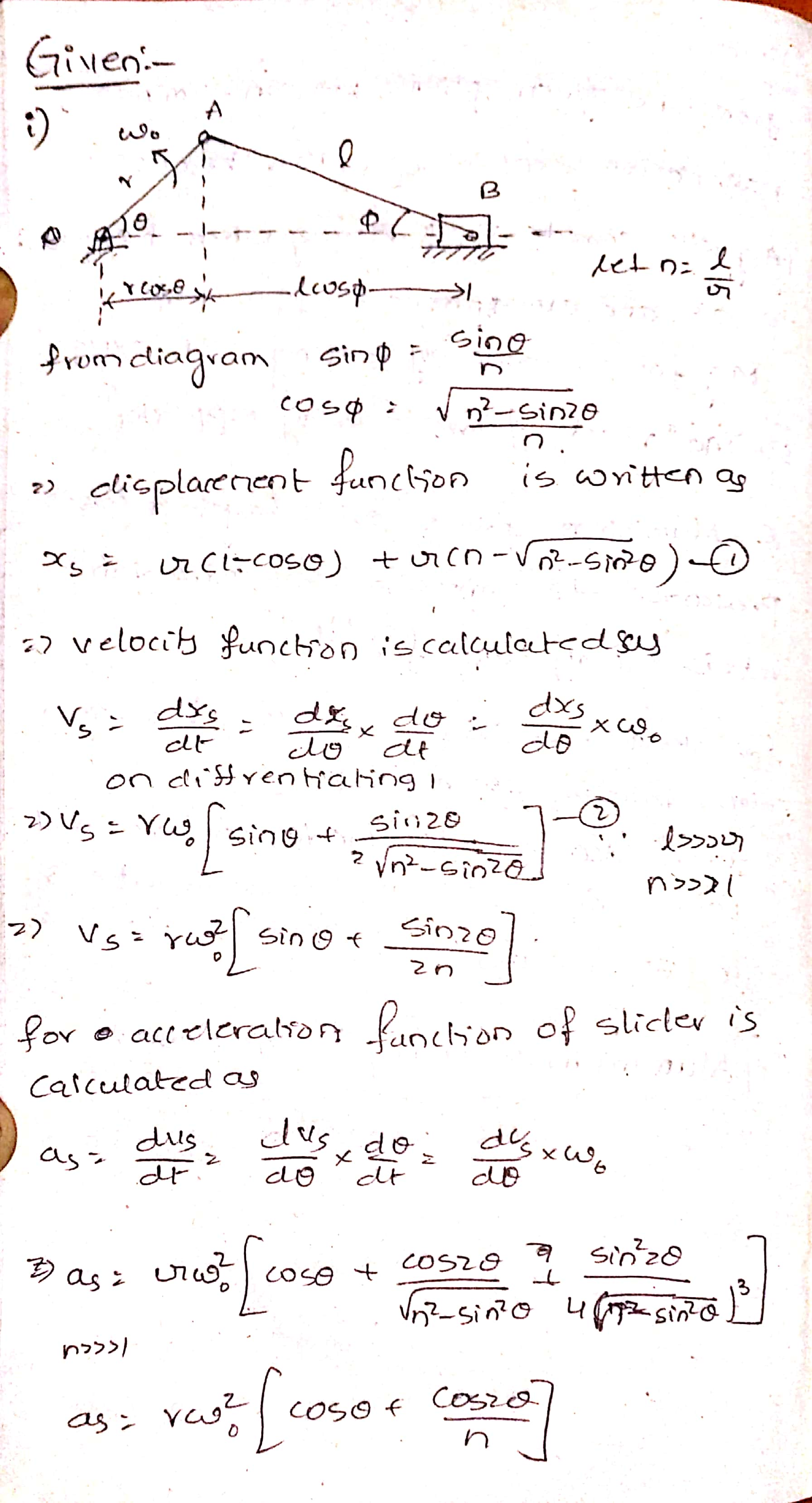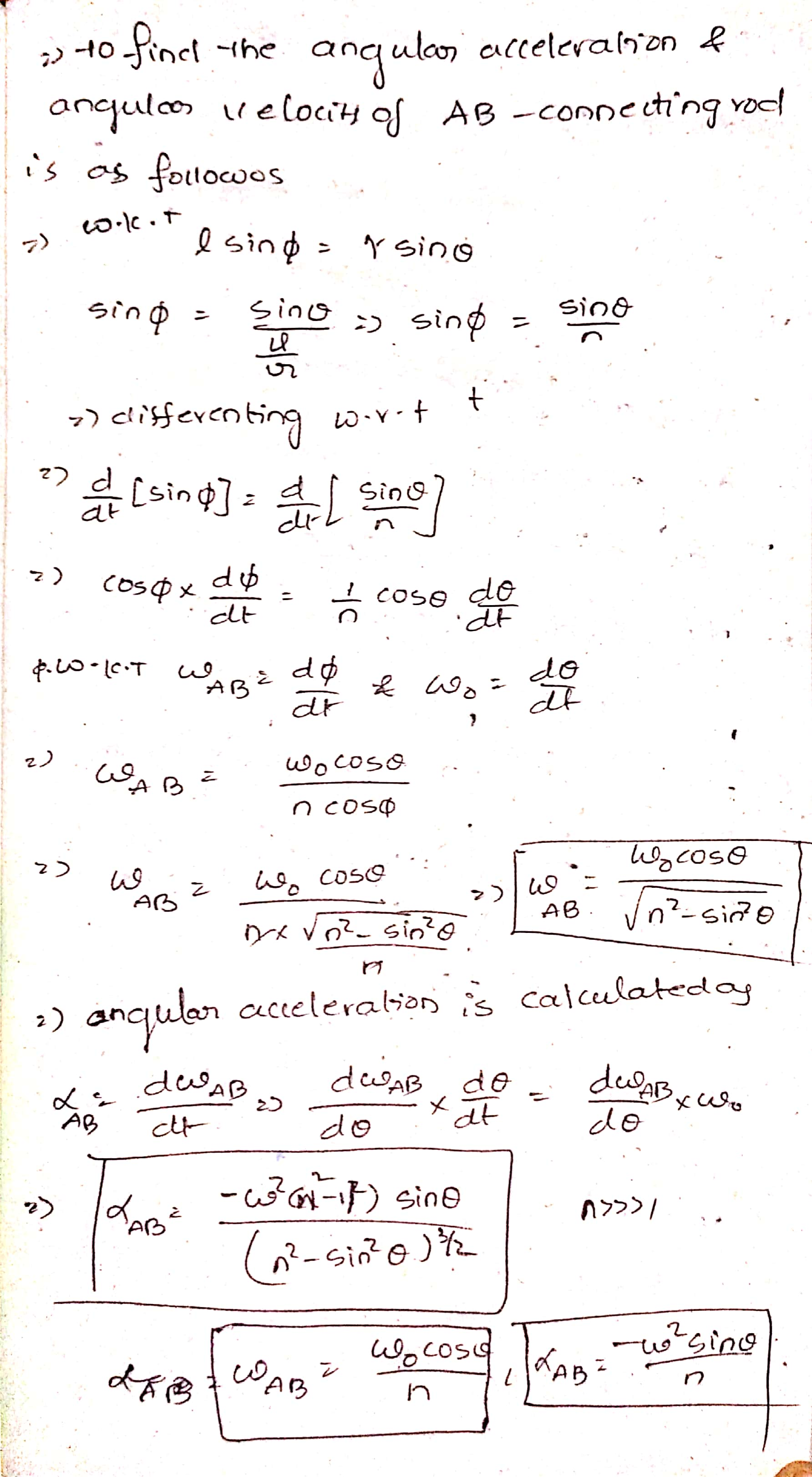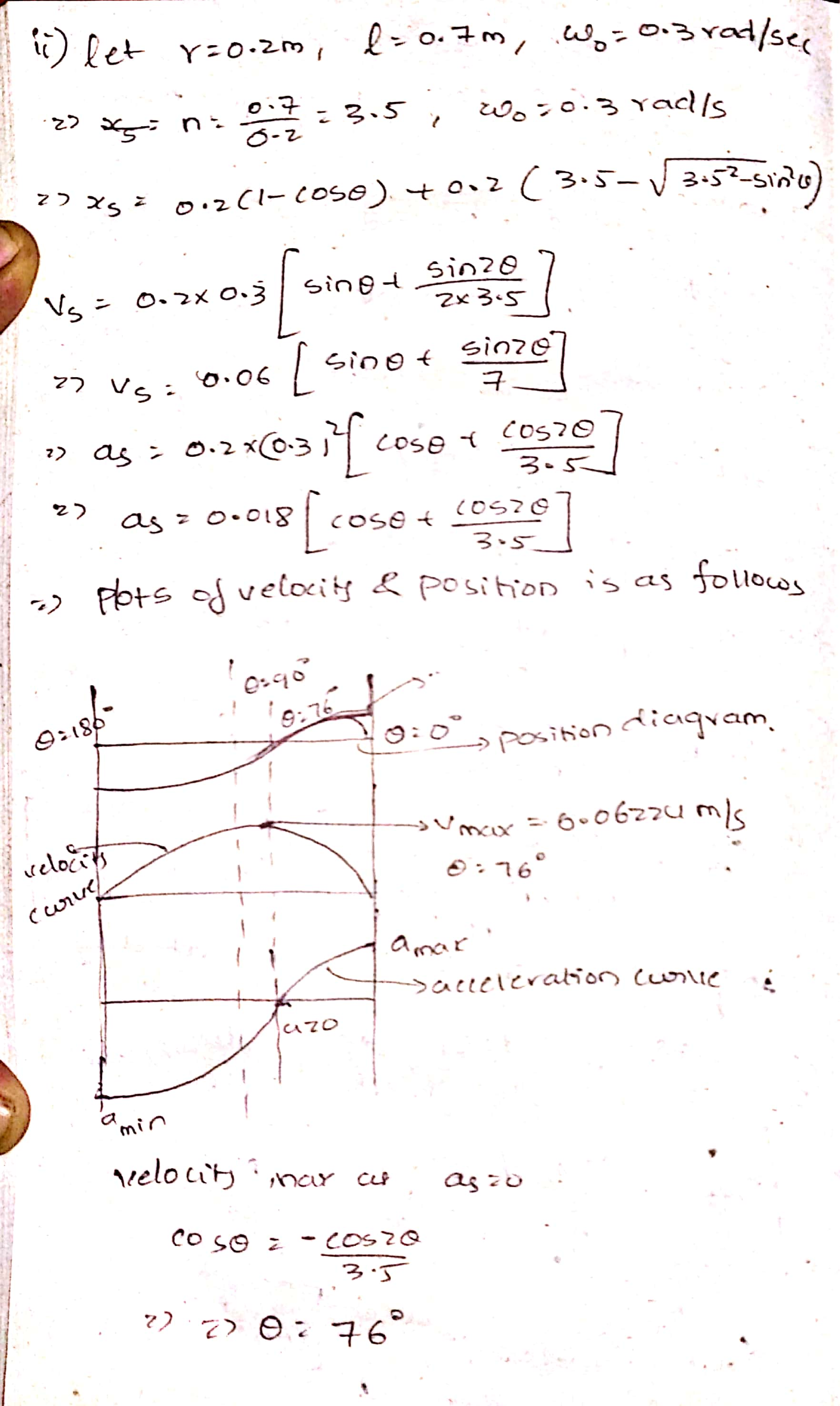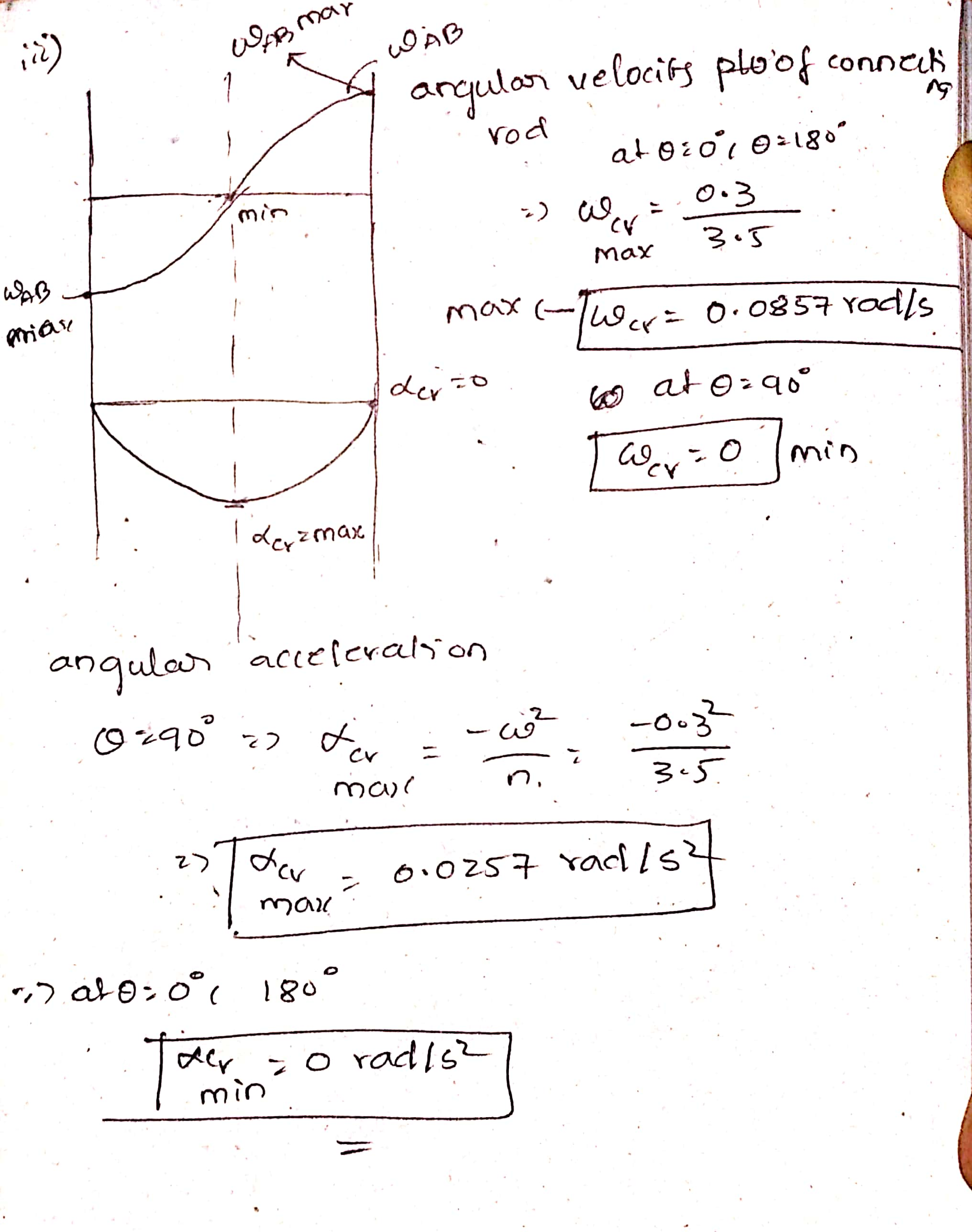#### Earn Coins

Coins can be redeemed for fabulous gifts.

Similar Homework Help Questions
• ### PROBLEM 2 (20 points) In the offset slider crank mechanism shown, the crank (AB) has a constant angular velocity of 10 rad/s, counterclockwise (A) Draw (clearly) the two limiting positions of the...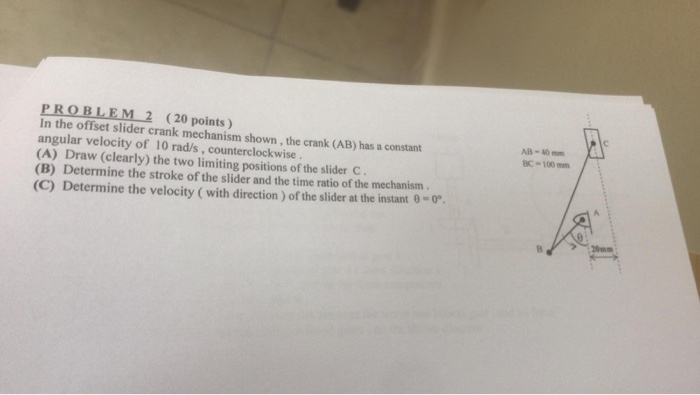PROBLEM 2 (20 points) In the offset slider crank mechanism shown, the crank (AB) has a constant angular velocity of 10 rad/s, counterclockwise (A) Draw (clearly) the two limiting positions of the slider c (B) Determine the stroke of the slider and the time ratio of the mechanism (C) Determine the velocity ( with direction) of the slider at the instant 8- AB- 40 mm C-100mm PROBLEM 2 (20 points) In the offset slider crank mechanism shown, the crank (AB)...

• ### QUESTION 1 A slider crank linkage is shown in figure 3 below. The angular velocity and...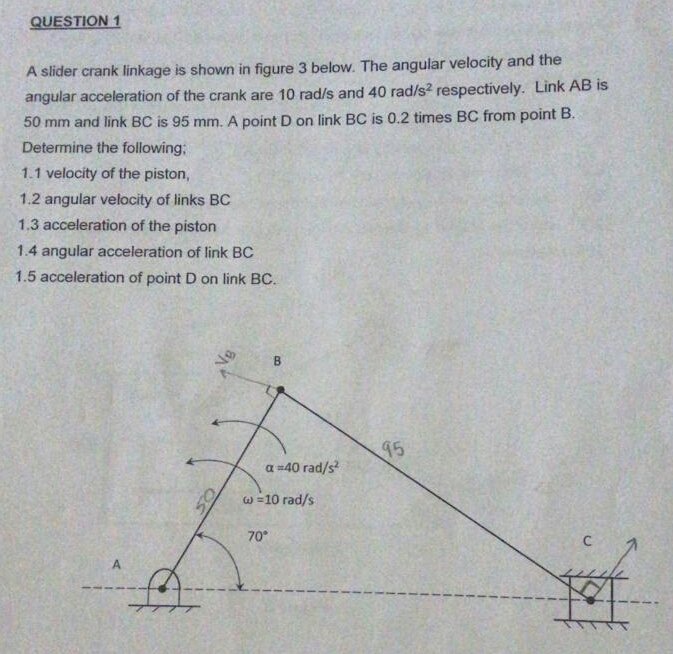QUESTION 1 A slider crank linkage is shown in figure 3 below. The angular velocity and the angular acceleration of the crank are 10 rad/s and 40 rad/s2 respectively. Link AB is 50 mm and link BC is 95 mm. A point D on link BC is 0.2 times BC from point B Determine the following; 1.1 velocity of the piston, 1.2 angular velocity of links BC 1.3 acceleration of the piston 1.4 angular acceleration of link BC 1.5 acceleration...

• ### Problem 3.3 In the engine system shown, the crank AB has a constant counterclockwise angular velocity of 200 rad/s...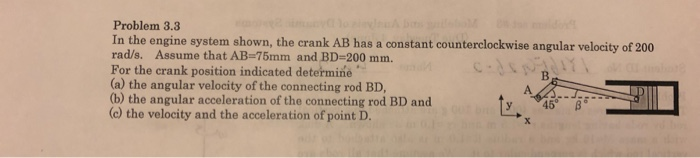Problem 3.3 In the engine system shown, the crank AB has a constant counterclockwise angular velocity of 200 rad/s. Assume that AB-75mm and BD-200 mm. For the crank position indicated determine (a) the angular velocity of the connecting rod BD, (b) the angular acceleration of the connecting rod BD and (c) the velocity and the acceleration of point D. Problem 3.4 A thin homogeneous wire with Determine the period of small oscillation if the wire (a) is suspended as shown,...

• ### DYNAMICS An internal combustion engine slider-crank mechanism is shown in the figure. Crank AB rotates in...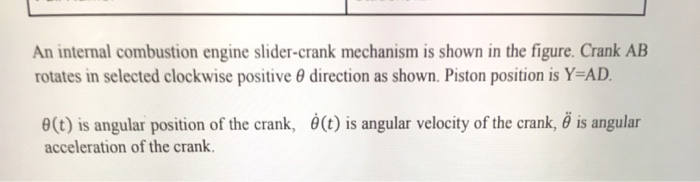DYNAMICS An internal combustion engine slider-crank mechanism is shown in the figure. Crank AB rotates in selected clockwise positive direction as shown. Piston position is Y=AD. e(t) is angular position of the crank, ó(t) is angular velocity of the crank, ő is angular acceleration of the crank, y Р D 150 mm А e B - - X 50 mm 5) Crank AB rotates starting from rest with a constant angular acceleration of 0.25 rad/sec? ( = 0.25 rad/sec? )...

• ### An internal combustion engine slider-crank mechanism is shown in the figure. Crank AB rotates in selected...An internal combustion engine slider-crank mechanism is shown in the figure. Crank AB rotates in selected clockwise positive direction as shown. Piston position is Y=AD. e(t) is angular position of the crank, ó(t) is angular velocity of the crank, ő is angular acceleration of the crank, 4) Crank AB rotates starting from rest with a constant angular acceleration of 0.25 rad/sec? ( = 0.25 rad/sec² ) clockwise in positive 0 direction as shown Perform computer simulations using above formulas to...

• ### determine the angular velocity of the crank of the slider-crank mechanism shown below FIGURE 2.58 YAB...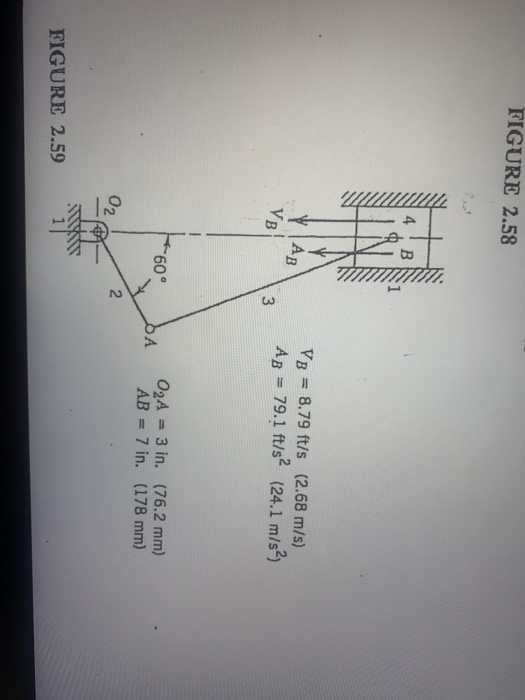determine the angular velocity of the crank of the slider-crank mechanism shown below FIGURE 2.58 YAB YB VB = 8.79 ft/s (2.68 m/s) Ag = 79.1 ft/s? (24.1 m/s2 3 БА 02A = 3 in. (76.2 mm) AB = 7 in. (178 mm) S2 FIGURE 2.59

• ### In a slider crank mechanism at the given instant member AB (crank) has uniform angular motion...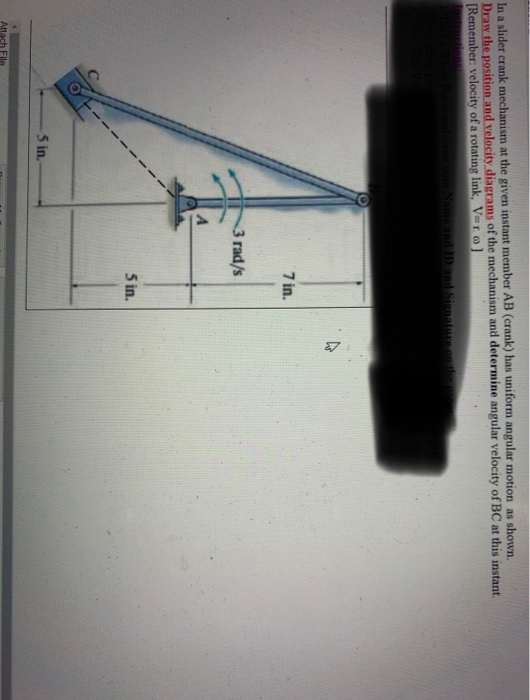In a slider crank mechanism at the given instant member AB (crank) has uniform angular motion as shown. Draw the position and velocity diagrams of the mechanism and determine angular velocity of BC at this instant. [Remember: velocity of a rotating link, Vero] Name and ID and Sienature on the 7 in. 3 rad/s Sin. 5 in. Attach File

• ### Question 4 (15 marks) The figure below shows a slider-crank mechanism. Link AB is driven with...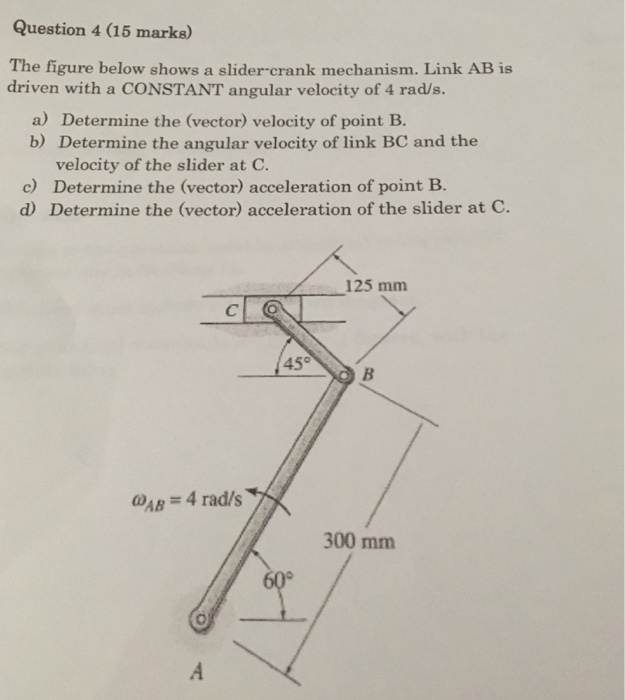Question 4 (15 marks) The figure below shows a slider-crank mechanism. Link AB is driven with a CONSTANT angular velocity of 4 rad/s. a) Determine the (vector) velocity of point B. b) Determine the angular velocity of link BC and the velocity of the slider at C. c) Determine the (vector) acceleration of point B. d) Determine the (vector) acceleration of the slider at C. 125 mm MAB = 4 rad/s 300 mm 600

• ### Crank OA rotates with a constant counterclockwise angular velocity of 13.6 rad/s. Determine the angular acceleration...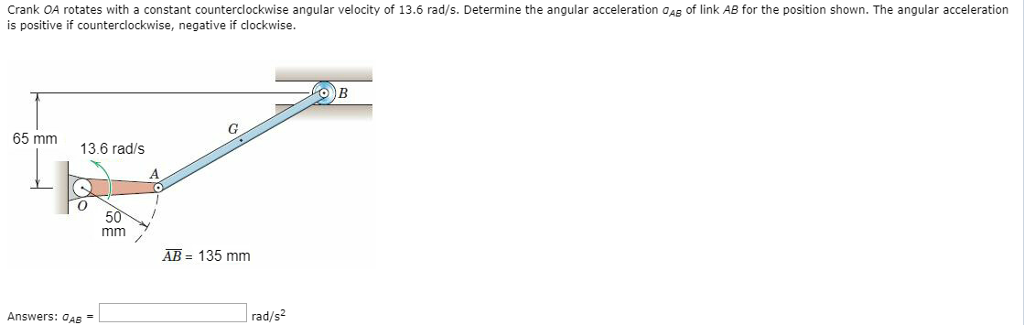Crank OA rotates with a constant counterclockwise angular velocity of 13.6 rad/s. Determine the angular acceleration oAB of link AB for the position shown. The angular acceleration is positive if counterclockwise, negative if clockwise 65 mm 13.6 radls 0 AB 135 mm Answers: OAB rad/s2

• ### ine given 4) In the figure aside, an in-line slider- crank mechanism is shown. a) Calculate the velocity of the cou...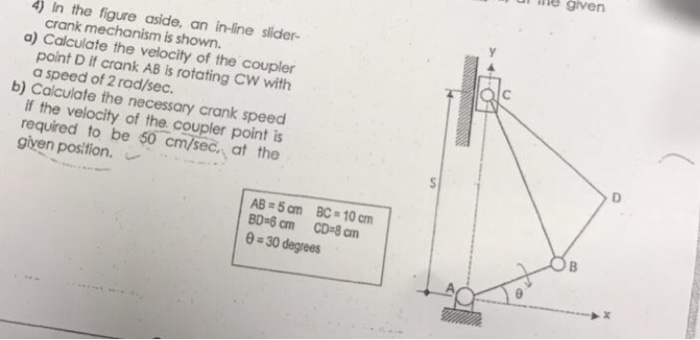ine given 4) In the figure aside, an in-line slider- crank mechanism is shown. a) Calculate the velocity of the coupler point D if crank AB is rotating CW wilth a speed of 2 rad/sec b) Calculate the necessary crank if the velocity of the coupler point is required to be 50 cm/sec, at the given position. speed AB 5am BC-10cm 0 30 degrees ine given 4) In the figure aside, an in-line slider- crank mechanism is shown. a) Calculate...